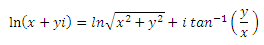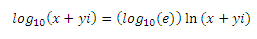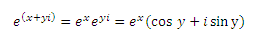Engineering Log functions with complex numbers

Excel Function IMLN

The IMLN() function is used to determine the natural logarithm of the complex number.

The natural logarithm is the log value with base e.

The logarithm of complex number includes the sum of logarithm of absolute value and logarithmComplex Number1 IMLN Formula 2+5i 1.68364791499324+1.19028994968253i =IMLN(L4) 4+2.3i 1.52911873945269+0.52183427981441i =IMLN(L5) 6+5.7i 2.11334387460491+0.759762754875771i =IMLN(L6) 1.4+8i 2.0945242488457+1.39755066034253i =IMLN(L7) 1.7+11i 2.40969703756382+1.41746393839657i =IMLN(L8)

Excel Function IMLOG10

The IMLOG10() function is used to determine the logarithm of a complex number with base-10.

The inverse of log base 10 is 10^x.

The formula to calculate log base 10 of a complex number x+iy is as given below:Complex Number1 IMLOG10 Formula 2+5i 0.731198998949478+0.516936357012023i =IMLOG10(L19) 4+2.3i 0.664087830719161+0.226629748191356i =IMLOG10(L20) 6+5.7i 0.917813583104949+0.32996077199816i =IMLOG10(L21) 1.4+8i 0.909640323486241+0.606948539967007i =IMLOG10(L22) 1.7+11i 1.04651812647258+0.615596766742483i =IMLOG10(L23)

Excel Function IMLOG2

The IMLOG10() function is used to determine the logarithm of a complex number with base-2.

The inverse of log base 2 is 2^x.

The formula to calculate log base 2 of a complex number x+iy is as given below:Complex Number1 IMLOG2 Formula 2+5i 2.42899049756379+1.71722540762696i =IMLOG2(L34) 4+2.3i 2.20605202233878+0.752847727654114i =IMLOG2(L35) 6+5.7i 3.04891072758557+1.09610595871141i =IMLOG2(L36) 1.4+8i 3.02175974683137+2.01623940706727i =IMLOG2(L37) 1.7+11i 3.47645796613816+2.04496819456368i =IMLOG2(L38)

Excel Function IMPOWER

The IMPOWER() function is used to determine the value of a complex number raised to a given power.

The power value can be floating number as well and the complex number is multiplied by itself

for the given power values times.

 Complex Number1 Power IMPOWER Formula 2+5i 2 -21+20i =IMPOWER(L48,M48) 4+2.3i 3 0.520000000000033+98.233i =IMPOWER(L49,M49) 6+5.7i 5 -30734.397-23716.55943i =IMPOWER(L50,M50) 1.4+8i 3 -266.056-464.96i =IMPOWER(L51,M51) 1.7+11i 2.1 -155.508850585153+25.8813355491157i =IMPOWER(L52,M52)

Excel Function IMEXP

The IMEXP() function returns the exponential of the complex number.

The resultant value is the e raised to the power of the given complex number.

The formula is as shown below:Complex Number1 IMEXP Formula 2+5i 2.09599580153305-7.08554526011231i =IMEXP(L61) 4+2.3i -36.3774381733222+40.7141250549222i =IMEXP(L62) 6+5.7i 336.747171700623-222.162404044057i =IMEXP(L63) 1.4+8i -0.590031732276589+4.01204552890464i =IMEXP(L64)

It should be noted that, the value of i^2 is -1 and is fixed.